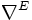# Geometric Dirac structure

A geometric Dirac structure on a Riemannian manifold$(M,g)$ is a Dirac structure where the connection on the manifold is the Levi-Civita connection. Explicitly, it is a quadruple$(E,c,\nabla^E,\nabla^M)$ where:
•$E$ is a Hermitian vector bundle on$M$
•$c: T^*(M) \to End(E)$ is a self-adjoint Clifford structure
•$\nabla^M$ is the Levi-Civita connection on$M$
•$\nabla^E$ is a Hermitian connection on$E$ compatible with the Clifford multiplication